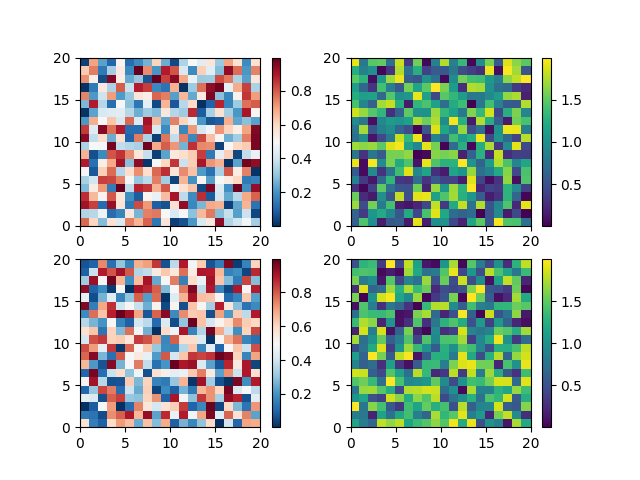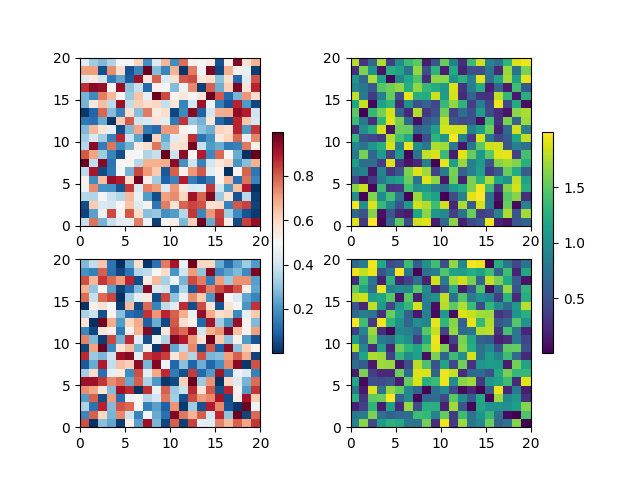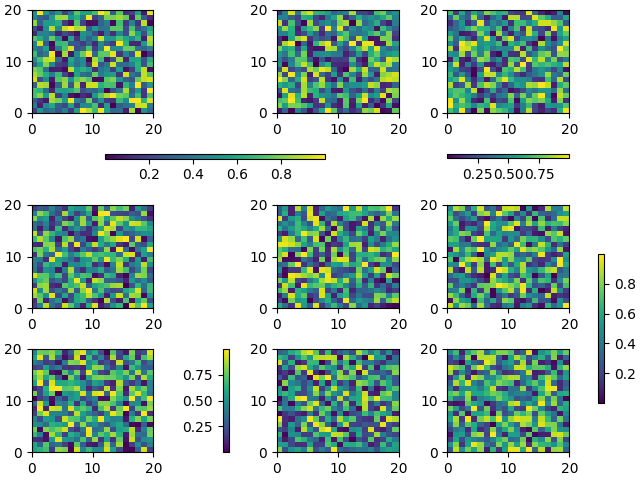# Placing Colorbars¶

Colorbars indicate the quantitative extent of image data. Placing in a figure is non-trivial because room needs to be made for them.

The simplest case is just attaching a colorbar to each axes:

import matplotlib.pyplot as plt
import numpy as np

fig, axs = plt.subplots(2, 2)
cm = ['RdBu_r', 'viridis']
for col in range(2):
for row in range(2):
ax = axs[row, col]
pcm = ax.pcolormesh(np.random.random((20, 20)) * (col + 1),
cmap=cm[col])
fig.colorbar(pcm, ax=ax)
plt.show()The first column has the same type of data in both rows, so it may be desirable to combine the colorbar which we do by calling Figure.colorbar with a list of axes instead of a single axes.

fig, axs = plt.subplots(2, 2)
cm = ['RdBu_r', 'viridis']
for col in range(2):
for row in range(2):
ax = axs[row, col]
pcm = ax.pcolormesh(np.random.random((20, 20)) * (col + 1),
cmap=cm[col])
fig.colorbar(pcm, ax=axs[:, col], shrink=0.6)
plt.show()Relatively complicated colorbar layouts are possible using this paradigm. Note that this example works far better with constrained_layout=True

fig, axs = plt.subplots(3, 3, constrained_layout=True)
for ax in axs.flat:
pcm = ax.pcolormesh(np.random.random((20, 20)))

fig.colorbar(pcm, ax=axs[0, :2], shrink=0.6, location='bottom')
fig.colorbar(pcm, ax=[axs[0, 2]], location='bottom')
fig.colorbar(pcm, ax=axs[1:, :], location='right', shrink=0.6)
fig.colorbar(pcm, ax=[axs[2, 1]], location='left')

plt.show()Total running time of the script: ( 0 minutes 1.124 seconds)

Keywords: matplotlib code example, codex, python plot, pyplot Gallery generated by Sphinx-Gallery